NCERT Solutions: Number System (Exercise 1.1)

# Number System (Exercise 1.1) NCERT Solutions - Mathematics (Maths) Class 9

Q1. Is zero a rational number? Can you write it in the form p/q where p and q are integers and q ≠ 0?
Ans: We know that a number is said to be rational if it can be written in the form p/q , where p and q are integers and q

Taking the case of '0',

Yes, zero is a rational number.
0 =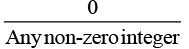Example :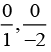Since it satisfies the necessary condition, we can conclude that 0 can be written in the p/q form, where q can either be positive or negative number.
Hence, 0 is a rational number.

Q2. Find six rational numbers between 3 and 4.
Ans: We can find any number of rational numbers between two rational numbers. There are infinite rational numbers between 3 and 4.

• First of all, we make the denominators same by multiplying or dividing the given rational numbers by a suitable number.
• If denominator is already same then depending on number of rational no. we need to find in question, we add one and multiply the result by numerator and denominator.

As we have to find 6 rational numbers between 3 and 4, we will multiply both the numbers, 3 and 4, with 6+1 = 7 (or any number greater than 6)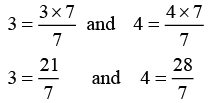We can choose 6 rational numbers as: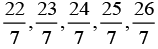and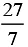Q3. Find five rational numbers between 3/4 and 4/5.
Ans: There are infinite rational numbers between 3/5 and 4/5.

Since we make the denominator same first, then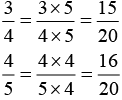To find out 5 rational numbers between 3/5 and 4/5, we will multiply both the numbers 3/5 and 4/5

with 5+1=6 (or any number greater than 5)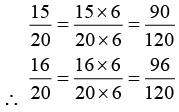∴ Five rational numbers between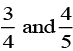are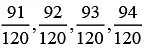and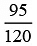Q4. State whether the following statements are true or false. Give reasons for your answers.
(i) Every natural number is a whole number.
Ans: True
Natural Numbers: Natural numbers are set of numbers that contain numbers from 1 to infinity.  Set of natural numbers is represented as N= {1, 2, 3…….}.
Whole numbers: Numbers starting from 0 to infinity (without fractions or decimals)
Set of  Whole numbers  is represnted as W= 0,1,2,3….
As all the natural numbers comes in the set of whole numbers. Hence, every natural number is a whole number.

(ii) Every integer is a whole number.
Ans: False
Integers: Integers are set of numbers that contain positive, negative and 0; excluding fractional and decimal numbers.
i.e., integers = {…-4,-3,-2,-1,0,1,2,3,4…}
Whole numbers: Numbers starting from 0 to infinity (without fractions or decimals)
i.e., Whole numbers = 0,1,2,3….
Hence, we can say that integers include whole numbers as well as negative numbers.
Every whole number is an integer; however, every integer is not a whole number.

(iii) Every rational number is a whole number.
Ans: False
Rational numbers: All numbers in the form p/q, where p and q are integers and q ≠ 0.
i.e., Rational numbers = 0, 19/30 , 2, 9/-3, -12/7…
Whole numbers: Numbers starting from 0 to infinity (without fractions or decimals)
i.e., Whole numbers = 0,1,2,3….
Hence, we can say that integers include whole numbers as well as negative numbers.
Every whole number is rational, however, every rational number is not a whole number.

The document Number System (Exercise 1.1) NCERT Solutions | Mathematics (Maths) Class 9 is a part of the Class 9 Course Mathematics (Maths) Class 9.
All you need of Class 9 at this link: Class 9

## Mathematics (Maths) Class 9

42 videos|378 docs|65 tests

## FAQs on Number System (Exercise 1.1) NCERT Solutions - Mathematics (Maths) Class 9

 1. What is the Number System?Ans. The Number System is a way to represent and manipulate numbers. It includes various types of numbers such as natural numbers, whole numbers, integers, rational numbers, and irrational numbers.
 2. What are Natural Numbers?Ans. Natural numbers are positive integers starting from 1 and going up to infinity. They are commonly used for counting and are denoted by the symbol 'N'.
 3. What is the difference between Whole Numbers and Integers?Ans. Whole numbers include all the natural numbers along with zero. On the other hand, integers include all the whole numbers as well as their negatives. In other words, integers include both positive and negative numbers.
 4. How do we classify numbers as Rational or Irrational?Ans. Rational numbers can be expressed as fractions, i.e., they can be written in the form p/q where p and q are integers and q is not equal to zero. Irrational numbers, on the other hand, cannot be expressed as fractions and their decimal representation goes on forever without repeating.
 5. Why are Number Systems important in mathematics?Ans. Number Systems are the foundation of mathematics and are used in various mathematical operations and calculations. They help us understand the properties and relationships of numbers, which are essential in solving equations, analyzing data, and making mathematical predictions.

## Mathematics (Maths) Class 9

42 videos|378 docs|65 testsExplore Courses for Class 9 examSignup to see your scores go up within 7 days! Learn & Practice with 1000+ FREE Notes, Videos & Tests.
10M+ students study on EduRev
Track your progress, build streaks, highlight & save important lessons and more!
Related Searches

,

,

,

,

,

,

,

,

,

,

,

,

,

,

,

,

,

,

,

,

,

;